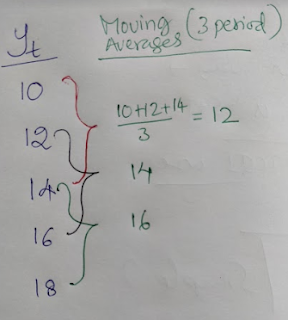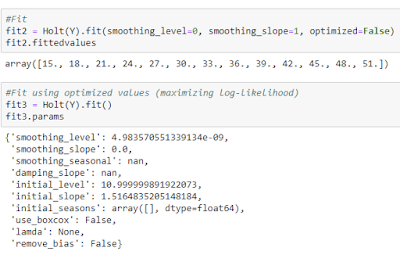### Exponential Smoothing Techniques

In this post, let us explore:

• Moving Averages
• Single Exponential Smoothing
• Double Exponential Smoothing
• Triple Exponential Smoothing

### Moving Averages

Moving Averages help in smoothing the data. It reduces the effect of irregular variations in time series data.Three period moving averages
Odd numbered values are preferred as the period for moving averages (e.g. 3 or 5) because the average values is centred. If we want to calculate moving averages with even number of observations (such as 2 or 4), then we have to take average of moving averages to centre the values.

### Single Exponential Smoothing

Ft+1 = αYt+(1-α)Ft
where α is smoothing constant (lies between and 0 and 1).

The following picture shows how to forecast using single exponential smoothing technique with α = 1. For the first time period, we cannot forecast (left blank). For the second period (t=2), we take the actual value for the previous period as the forecast (46 in this case).

Some use the average of values of first few observations instead (average of let us say first four observations: 46,56,54 and 43). Actual forecasting work starts from the third time period (t=3).

So how to choose the appropriate value for α? This depends on data. One way is to estimate different forecast series using different α values and compute the forecast errors. Use the α value which has lowest forecast error. Generally a lower α value (0.01 to 0.3) is chosen.

Larger the value of α, higher the weights given to recent observations. Notice how α=1 series is nothing but a lagged actual series (Yt) [which is called Naive Method in time series], whereas α=0 means flat forecast series [called Average method if the initial value is based on average values of all observations].

But in presence of trend, single exponential smoothing is inadequate as shown in the graph below (link).

### Double Exponential Smoothing (Holt's method)

This method involves computing level and trend components. Forecast is the sum of these two components. As shown in the below picture, equation for level component is similar to the previously discussed single exponential smoothing.

As in the previous case, α is smoothing constant lies between 0 and 1. The second equation, trend component, as the name suggests captures the trend. The trend-smoothing constant, γ, also lies between 0 and 1. Finally we sum these two components to arrive at the forecasts.

In the excel sheet shown below, I used α = 0.1 and γ = 0.3.

Initial values
• For the first row, there is no forecast.
• In the second row, i.e. Sis generally same as the Y1 value (12 here). The initial value of b2 can be calculated in three ways (link). I have taken the difference between Y2 and Y1 (15-12=3). The first forecast F2 is same as Y1 (which is same as S2).
• I have described how arrived at the values in the third row in a picture given below this excel screenshot.

In some books and in Python, β is used to denote trend-smoothing constant.
I suggest you to read this blogpost for more on this.

#### Double Exponential Smoothing (Holt's method) in Python

These are two hyperparameters which we can control:

• smoothing_level = α = smoothing constant
• smoothing_slope = β ( in our above example we used gamma) = trend-smoothing constantAutomatically optimize alpha and beta values

### Triple Exponential Smoothing (Holt-Winter's method)

Double exponential smoothing works fine when there is trend in time series, however it fails in presence of seasonality. Additionally, Triple Exponential Smoothing includes a seasonal component as well. It is also called Holt-Winters method. There are two models under these:

• Multiplicative Seasonal Model

For detailed methodology you can go through this excellent paper. In a nutshell,

### Summary

In this post, we have seen the concept and examples of

• Moving Averages
• Single Exponential Smoothing
• Double Exponential Smoothing
• Triple Exponential Smoothing
If you have any questions or suggestions, do share. I will be happy to interact.

References: